Beams Index

## Curved beams

Introduction

The beam theory can also be applied to curved beams allowing the stress to be determined for shapes including crane hooks and rings.  When the dimensions of the cross section are small compared to the radius of curvature of the longitudonal axis the bending theory can be relatively accurate.  When this is not the case even using the modified Bernoulli-Euler only provides approximate solutions

Symbols
 ε = strain e = eccentricity (r c - r n)   (m) c c = Distance from centroid axis to inner surface. (m) c i = Distance from neutral axis to inner surface. (m) c o = Distance from neutral axis to outer surface. (m) dφ= Surface rotation resulting from bending stress σ = stress (N/m2) E = Young's Modulus = σ /e (N/m2) y = distance of surface from neutral surface (m). r n = Radius of neutral axis (m). r c = Radius of centroid (m). r = Radius of axis under consideration (m). I = Moment of Inertia (m4 - more normally cm4) Z = section modulus = I/y max(m3 - more normally cm3)
Theory

The sketch below shows a curved member subject to a bending moment M.   The neutral axis r n and the centroid r c are not the same.
This is the primary difference between a straight beam and a curved beam.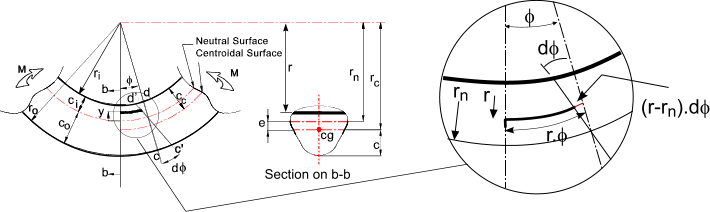>

The strain at a radius r =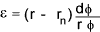The strain is clearly 0 when r = at the neutral axis and is maximum when r = the outer radius of the beam (r = r o )
Using the relationship of stress/strain = E the normal stress is simply.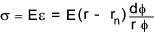The location of the neutral axis is obtained from summing the product of the normal stress and the area elements over the whole area and equating to 0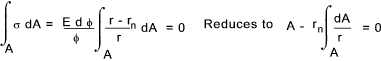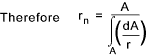Neutral Axis for a Rectangular Section..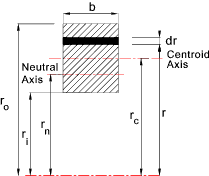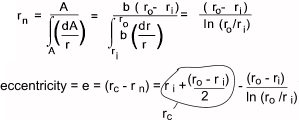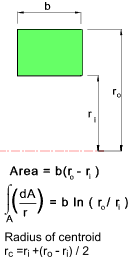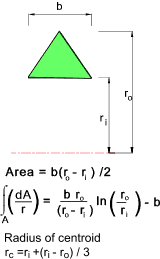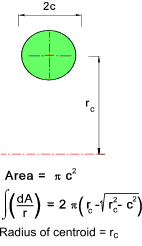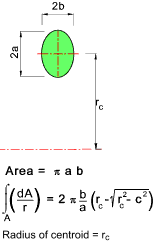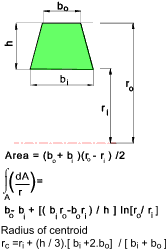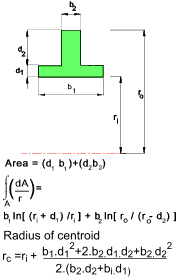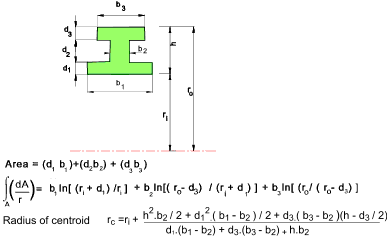Curved Beam in Bending

The stress resulting from an applied bending moment is derived from the fact that the resisting moment is simple the integral over the whole section of the moment arm from the neutral axis (y) multiplied by σdA (= dF).   Moment equilibrium is achieved if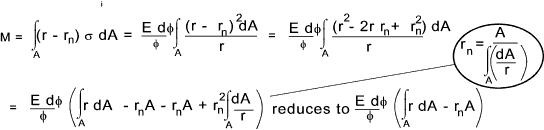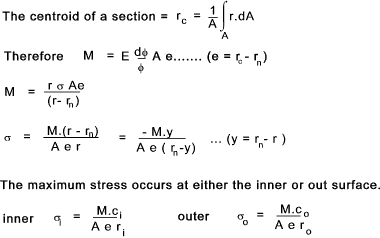The curved beam flexure formula is in reasonable agreement for beams with a ratio of curvature to beam depth of rc/h of > 5  (rectangular section).   As the beam curvature/depth radius increases the difference between the maximum stress calculated by curved beam formula and the normal beam formula reduces.   If the ratio is about 8 then a maximum stress error of only about 5% results from using the straight beam formulae.

Note:
The above equations are valid for pure bending.  In the more normal cases of e.g crane hooks, the bending moment is due to forces acting on one side of the section under consideration.  The bending moment, in this case has to be taken about the centroidal axis , not the neutral axis and the additional tensile or compressive stresses have to be considered to obtain the resultant stresses on the section. ( see example below).

Example Hook Calculation

The hook is lifting a load of 25000N.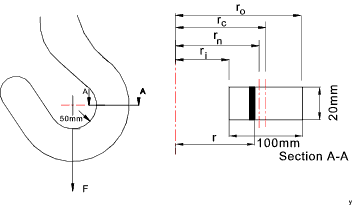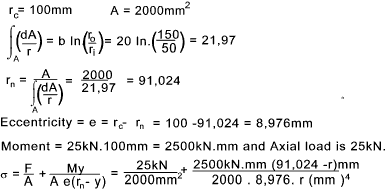The stress values plotting against r are shown below: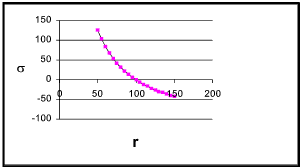The tensile stress at the inner surface is calculated at 126.76 N/mm2 and the compressive stress at the outer surface is calculated at -42,25 N/mm2...This section profile results in a tensile stress three times greater than the compressive stress.  A more efficient section with the stresses balanced would result from having a wider inner section and a thinner outer section.

 Sites Providing Relevant Information Thick curved beams...U.of West Australia ..Comprehensive Notes -excellent Beams composite curve, unsymmetrical...Washington U. Paper download Pure Bending...Lecture Notes including some notes on curved beams
Beams Index